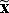# Variable Charts

All variable charts have two graphs on which the data are plotted, one of which monitors location (how close each subgroup is to the process average), while the other monitors dispersion (the amount of variation within successive subgroups). The names of the major variable charts reflect the particular statistics plotted on each of these two graphs. You will often find them referred to by their shorthand names (given in brackets in the list below). The most commonly used are:

• The Mean & Range (& R) Chart: the most commonly used chart of all, this chart records the mean (simple average) of each subgroup together with the range (the difference between the highest and lowest values). It is very sensitive to changes in the process and requires little calculation, hence its popularity.
• The Mean & Standard Deviation (& s) Chart: this is similar to the previous chart except it uses the standard deviation instead of the range as the measure of subgroup dispersion. The standard deviation is a more efficient indicator of process variability than the range and is more reliable with larger subgroups. However, the calculation required is much more complicated than that for the range, therefore this type of chart is rarely used except in SPC software packages.
• The Median & Range (& R) Chart: this chart uses the median value of each subgroup as the measure of location. The median is the middle value of a set of numbers when they are ordered numerically. It is not as sensitive as the mean & range chart, but was devised because it requires almost no calculation. The sample values are written onto the chart in descending order so finding the median is simply a case of picking the value in the middle. The only calculation required is to subtract the bottom value from the top one to find the range.
• The Individual & Moving Range (XmR) Chart: although control charts were originally conceived to work with subgroups, this is not always possible (for example when data volumes are low, or when the original ordering of the data is unknown). In these situations, an XmR chart can be used to record the individual values, as opposed to a subgroup statistic such as the mean.

Later in this course, we will be looking in greater detail at the mean & range and the individual & moving range charts.